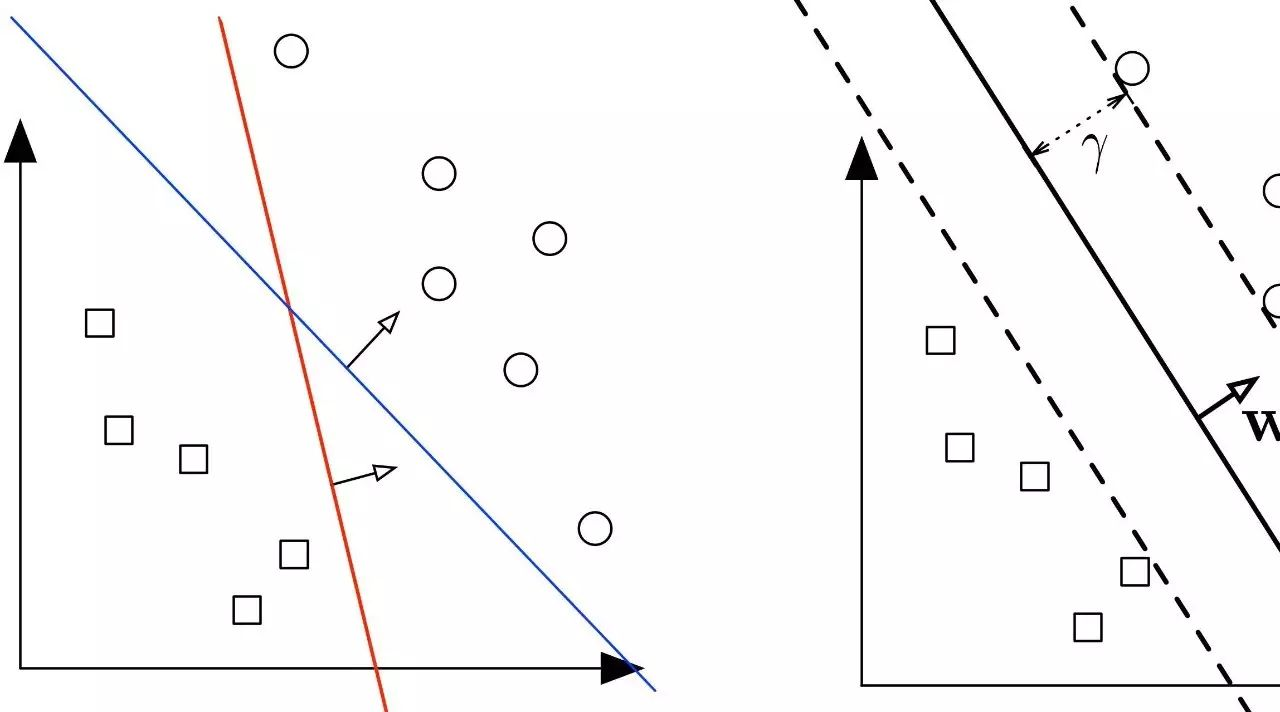## 【学习】(Python)SVM数据分类

2017 年 10 月 15 日 机器学习研究会Support Vector Machine (SVM) is a very powerful and flexible Machine Learning Model, capable of performing linear or nonlinear classificationregression, and even outlier detection. It is one of the most popular models in Machine Learning , and anyone interested in ML should have it in their toolbox. SVMs are particularly well suited for classification of complex but small or medium sized datasets. In this post we will explore SVM model for classification and will implement in Python.

## Linear SVM

Let’s say we have 2 classes of data which we want to classify using SVM as shown in the figure.

The 2 classes can clearly be seperated easily with a straight line (linearly seperable). The left plot shows the decision boundaries of 2 possible linear classifiers. An SVM model is all about generating the right line (called Hyperplane in higher dimension) that classifies the data very well. In the left plot, even though red line classifies the data, it might not perform very well on new instances of data. We can draw many lines that classifies this data, but among all these lines blue line seperates the data most. The same blue line is shown on the right plot. This line (hyperplane) not only seperates the two classes but also stays as far away from the closest training instances possible. You can think of an SVM classifier as fitting the widest possible street (represented by parallel dashed lines on the right plot) between the classes. This is called Large Margin Classification.

This best possible decision boundary is determined (or “supported”) by the instances located on the edge of the street. These instances are called the support vectors. The distance between the edges of “the street” is called margin.

### Soft Margin Classification

If we strict our instances be off the “street” and on the correct side of the line, this is calledHard margin classification. There are 2 problems with hard margin classification.

1) It only works if the data is linearly seperable.

2) It is quite sensitive to outliers.

In the above data classes, there is a blue outlier. And if we apply Hard margin classification on this dataset, we will get decision boundary with small margin shown in the left diagram. To avoid these issues it is preferable to to use more flexible model. The objective is to find a good balance between keeping the street as large as possible and limiting the margin violation (i.e., instances that end up in the middle of the street or even on the wrong side). This is called Soft margin classification. If we apply Soft margin classification on this dataset, we will get decision boundary with larger margin than Hard margin classification. This is shown in the right diagram.

## Nonlinear SVM

Although linear SVM classifiers are efficient and work surprisingly well in many cases, many datasets are not even close to being linearly seperable. One simple method to handle nonlinear datasets is to add more features, such as polynomial features and sometimes this can result in a linearly seperable dataset. By generating polynomial features, we will have a new feature matrix consisting of all polynomial combinations of the features with degree less than or equal to the specified degree. Following image is an example of using Polynomial Features for SVM.

## Kernel Trick

Kernel is a way of computing the dot product of two vectors